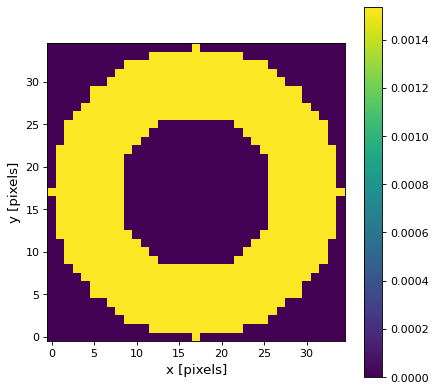# Ring2DKernel¶

class astropy.convolution.Ring2DKernel(radius_in, width, **kwargs)[source]

2D Ring filter kernel.

The Ring filter kernel is the difference between two Tophat kernels of different width. This kernel is useful for, e.g., background estimation.

Parameters
radius_innumber

Inner radius of the ring kernel.

widthnumber

Width of the ring kernel.

modestr, optional
One of the following discretization modes:
• ‘center’ (default)

Discretize model by taking the value at the center of the bin.

• ‘linear_interp’

Discretize model by performing a bilinear interpolation between the values at the corners of the bin.

• ‘oversample’

Discretize model by taking the average on an oversampled grid.

• ‘integrate’

Discretize model by integrating the model over the bin.

factornumber, optional

Factor of oversampling. Default factor = 10.

Examples

Kernel response:

import matplotlib.pyplot as plt
from astropy.convolution import Ring2DKernel
ring_2D_kernel = Ring2DKernel(9, 8)
plt.imshow(ring_2D_kernel, interpolation='none', origin='lower')
plt.xlabel('x [pixels]')
plt.ylabel('y [pixels]')
plt.colorbar()
plt.show()


(png, svg, pdf)